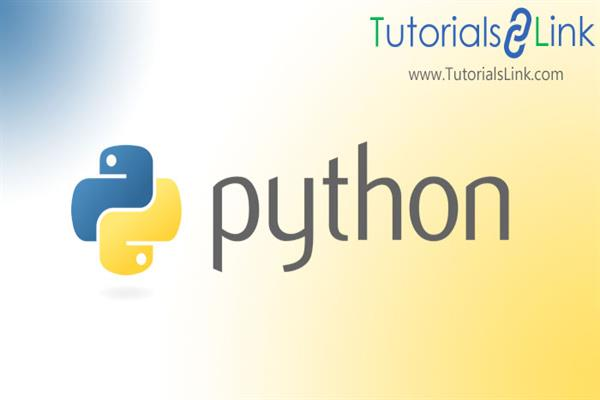Loading, please wait...# NumPy Array Filter, Join

1. What do you mean by filtering a NumPy Array?
Filtering an array means to getting some elements out of the original array and creating a newer one. In NumPy, the Boolean index list is used to filter an array. If the value at an index is True that element is appended in the filter array otherwise it’s is excluded from the filtered array.
we can easily create a NumPy filter in Python.

Syntax:

``filter(func, sequence)``

parameters:
Func: any function that checks if true or false.
Sequence: any sequence which we want to be filtered, it may be a set, lists, tuples of any iterators.

For example python code to demonstrate the working of filter() function:

``````£python code to filter even numbers out of the ndarray
#create empty filter array
filterarray=[]

#iterating
for num in arr:
if num %2 == 0:
filterarray.append(True)
else:
filterarray.append(False)

even_num_array = arr[filterarray]
print(filterarray)
#output:[False, False, True, True, True, False, True, True]
print(even_num_array)
#output:[90 56 76 78 98]``````

Note:  it is mostly used with lambda functions to separate lists, sets, or tuples.

Example:
Use of filter function with a lambda function

``````#function to find the intersection of two arrays
def intersection(arry1, arry2):
#resultant is holding the list of filtered array
resultant = list(filter(lambda x: x in arry1,arry2))
print(resultant)

if __name__ == "__main__":
my_arry1 =[3,67,89,56,87]
my_arry2 =[56,80,90,67,70]
intersection(my_arry1,my_arry2)

output:``````

2. Joining or Concatenating NumPy array:

Joining NumPy arrays means to put the content of one or more arrays in a single array. In SQL, we use keys for joining one or more tables, here we use axis to join one or more arrays in a single array.

Syntax:

``````numpy.concatenate((arr1,arr2,arr3,…..), axis=0, out=None)
``````

a1,a2… are the sequence of the array we pass must have the same shape, except in the dimension corresponding to the axis(first by default)
axis: it is an integer value, and optional too, by default it is 0.
out: optional

if we pass the sequence of arrays to concatenate() function, along with the axis.
Let’s see an example code on how we can do this.
a) Merging 1-D arrays.

Example: merging three 1-D NumPy arrays.

``````import numpy as np
my_arry1 =np.array([3,67,89,67,56,87])
my_arry2 =np.array([56,80 ,90,67,70])
my_arry3 =np.array([1,2,3,5,5])
concatenated_array=np.concatenate((my_arry1,my_arry2,my_arry3))
print(concatenated_array)

#output:[ 3 67 89 67 56 87 56 80 90 67 70 1 2 3 5 5]``````

b) Merging 2-D arrays.

Example: merging two 2-D NumPy arrays.

``````import numpy as np
my_arry1 =np.array([[3,7,89],[67,56,87]])
my_arry2 =np.array([[56,80,81],[90,97,70]])
concatenated_array=np.concatenate((my_arry1,my_arry2))
print(concatenated_array)

#output:
#[[ 3 7 89]
# [67 56 87]
# [56 80 81]
# [90 97 70]]
``````

c) Merging 2-D arrays along the axis.

Example: merging two 2-D NumPy arrays along the axis.

``````import numpy as np
my_arry1 =np.array([[3,7,89],[67,56,87]])
my_arry2 =np.array([[56,80,81],[90,97,70]])
concatenated_array=np.concatenate((my_arry1,my_arry2), axis=1)
print(concatenated_array)

#output:
#[[ 3 7 89 56 80 81]
# [67 56 87 90 97 70]]``````

d) Joining Arrays Using Stack functions.

Stacking is done along a new axis. It is like concatenating 2-D arrays along the second axis which would result in putting them one over other.

i). Stacking NumPy array along the default axis:

#stacking two NumPy along default axis i.e 0

``````import numpy as np
my_arry1 =np.array([3,7,89])
my_arry2 =np.array([56,80,81])
new_array=np.stack((my_arry1,my_arry2))
print(new_array)

#output:
# [[ 3 7 89]
# [56 80 81]]``````

ii). Stacking NumPy array along axis = 1:

#stacking two NumPy arrays along the axis =1.

``````import numpy as np
my_arry1 =np.array([3,7,89])
my_arry2 =np.array([56,80,81])
new_array=np.stack((my_arry1,my_arry2), axis=1)
print(new_array)

output:
#[[ 3 56]
# [ 7 80]
# [89 81]]``````

e) stacking arrays in sequence horizontally(column-wise):

hstack(tuple) function takes a sequence of arrays as a parameter and stacks it horizontally.

Note: the shape of the array may or may not same.

#stacking sequence of numpy arrays using hstack() function.

``````import numpy as np
my_arry1 =np.array([3,7,89])
my_arry2 =np.array([56,80,81])
my_arry3 =np.array([1,2,4,5])
new_array=np.hstack((my_arry1,my_arry2,my_arry3))
print(new_array)

#output: [ 3 7 89 56 80 81 1 2 4 5]``````

f) stacking arrays in sequence vertically (row-wise):

vstack(tuple) function takes a sequence of arrays as a parameter and stacks it vertically.
Note: the shape of the array must be the same along all but the first axis, 1-D array must have the same length.

stacking sequence of NumPy arrays along rows i.e vertically using vstack() function.

``````import numpy as np
my_arry1 =np.array([3,7,89,12])
my_arry2 =np.array([56,80,81,13])
my_arry3 =np.array([1,2,4,5])
new_array=np.vstack((my_arry1,my_arry2,my_arry3))
print(new_array)

#output:
[[ 3 7 89 12]
[56 80 81 13]
[ 1 2 4 5]]``````

g) stacking arrays in sequence depth wise:

dstack(tuple) function takes a sequence of arrays as a parameter and stacks it along the third axis. It stacks along with the height, which is the same as depth.

#stacking sequence of NumPy arrays depth-wise i.e along the third axis using dstack() function.

``````import numpy as np
my_arry1 =np.array([3,7,89,12])
my_arry2 =np.array([56,80,81,13])

new_array=np.dstack((my_arry1,my_arry2))
print(new_array)

#output: [[ 3 56]
[ 7 80]
[89 81]]
[[[ 3 56]
[ 7 80]
[89 81]
[12 13]]]

``````